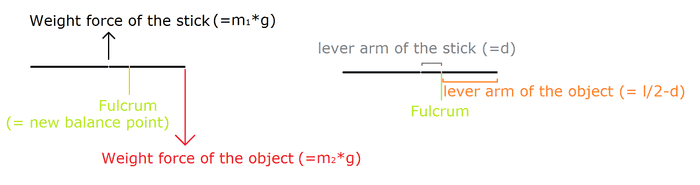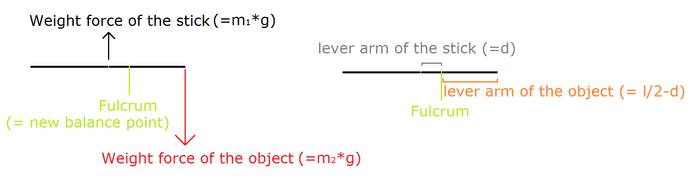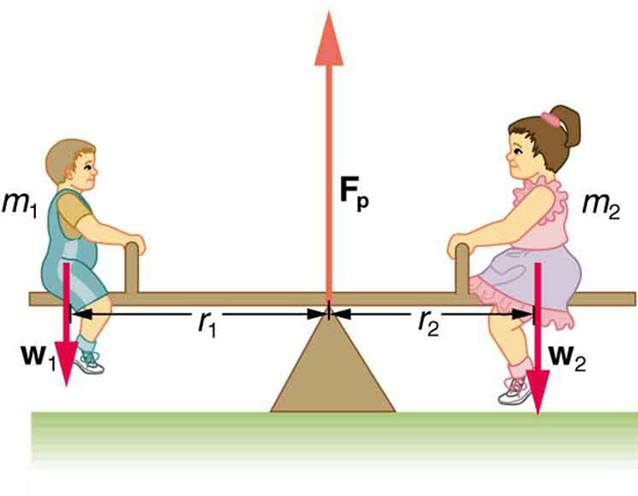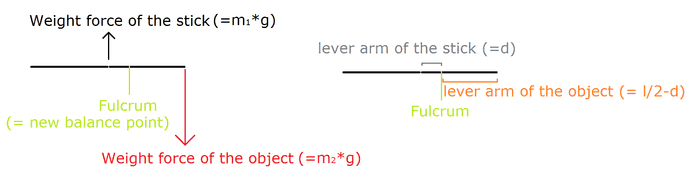# Equilibrium and Torque

There is a stick of known length l and known mass m1 with its balance point in the middle.
By placing an object of unknown mass m2 at the far end of the stick, the balance point moves towards the same end as the object by a distance of d.

Calculate m2.

Second, similar exercise:

Stick of known length l, unknown mass m1, balance point in the middle.
By placing an object of known mass m2 at a distance d1 away from one end of the stick, the balance point moves towards the same end as the object by a distance of d2.

Calculate m1.
-----
I already know how to solve each problem, but I don't know why they are solved the way they are.

By playing around with random equations and googling similar problems, the answer to the first problem is the following:

- Calculate Torque by the weight force of the stick using: m1*g*d
- Calculate Torque by the object using: m2*g*lever_arm
- The torques are opposed to one another since the system is in equilibrium, so solve the equation that way (m2 = m1*d/lever_arm)

What I don't understand:

Why can't we use the same "torque formula" for both? In other words, if I use m1*g*d=m2*g*lever_arm I'll get the right result, but if I use m1*g*d=m2*g*d2 (where d2 is l/2) I get the wrong result.

As far as I know, torque is generally defined by that Force*Distance equation, where "Distance" is the distance from the Force to the fulcrum, or rotation axis. Forces are m1*g and m2*g, and so far so good. So let's say I put the fulcrum at the original balance point of the stick: now d1 is = d, whereas d2 is (since the object is at the end of the stick) l/2.

The lever_arm is not l/2, but it's (l/2-d) or, in other terms, the lever_arm is the distance between the object and the new balance point.

I know that for the second exercise the same rule basically applies, and if I solve knowing the things I just wrote I will obtain:

m1*g*d = m2*g*lever_arm

Where lever_arm will be, again, the distance between the object and the new balance point (so it will be l/2-d1-d2).

By doing this, the result is correct.

But why? Why do we put the fulcrum/axis on the original balance point for the Force of the stick, but then we switch to the new balance point for the fulcrum/axis for the Force of the object? Why do we have to use two different torque equations (or at least they appear to be two separate ones), i.e. Torque = Force*Distance in one case, but Torque = Force*Lever_Arm = Force*(Length/2 - Distance) in the other case?

To me this seems like we choose two arbitrary axis of rotation: once in the middle of the stick, the other time at a distance d from the middle of the stick. It doesn't feel right to use two different "reference points" and then compare data depending on the two different reference points. But then again, that's how the result is correct. So what am I missing?

TSny
Homework Helper
Gold Member
Hi Alex. Welcome to PF!

There is only one formula to use: torque = force x lever arm.

Lever arm is distance from fulcrum to object if the object is localized at a point. The stick's mass is spread out along the stick. So, how do you find the lever arm for the stick? This is where the "center of gravity" (or center of mass) of the stick comes into play. This is the point where you can take the entire weight of the meter stick to be located for finding the distance between the fulcrum and the weight of the stick.

•Alex126
Thanks, but I still don't understand: why is it that in one case we use the center of the stick as the fulcrum (to calculate the torque by the stick), but in the other case (to calculate the "countertorque" by the object) we use the new balance point (center of the stick + d) as the fulcrum? If the fulcrum were the same for both, the equation for the first exercise (and something analogous for the second exercise) would either be (fulcrum in the middle of the stick, i.e. the original balance point) m1*d = m2*l/2, or (fulcrum in the new balance point) m1*0 = m2*(l/2-d). However, it's neither, but instead it's a combination of the two: m1*d = m2*(l/2-d).

So, again, why is the fulcrum the original balance point in one case, but the new balance point in the other case?

TSny
Homework Helper
Gold Member
When the problem says that the stick has "its balance point in the middle" it is just saying that the center of gravity of the stick is located at the midpoint of the stick. That means the stick would balance at that point if the fulcrum is at the middle of the stick and there are no other objects attached to the stick.

However, once an object is attached to the stick, then the fulcrum must be moved to a new location in order for the the system to balance. So, now there is no longer a fulcrum at the middle. The system balances with the new location of the fulcrum because the torque due to the weight of the object (force x lever arm) balances the torque due to the weight of the stick (force x lever arm). Here, both lever arms are measured from the new location of the fulcrum.

•Alex126
I'm still not quite sure about it, but maybe I found my problem. Correct me if I'm wrong:

- The fulcrum and balance point, aka the fulcrum and the center of mass of the stick, are in the same place when the system is isolated, i.e. before putting the item on the end of the stick. They are both in the middle of the stick. However, they are not the same thing, they just happen to be in the same place. As a matter of fact...
- ...after placing the object at one end of the stick, the fulcrum moves by a distance d, but the center of mass of the stick is still in its original position, that is to say it's still in the middle of the stick.
Small problem here: I thought the balance point and the center of mass were supposedly synonyms, but if what I just wrote is correct then fulcrum and balance point should be synonyms, while center of mass is not. Semantics...anyway, correct me if I'm wrong.

Therefore, if we draw the system it should look something like the following picture (the size of the vector arrows is random, but that's not the point):In this case, the result would be correct. Is this it?

Earlier I had thought that when the fulcrum moved, so did the balance point (obviously), but so did the center of mass as well. So, earlier, I thought that the weight force of the stick should have been represented not in the middle of the stick, but in correspondence with the new Fulcrum. Therefore, according to this process, the lever arm would have been =0, and could have only been =d if the fulcrum was still considered in the middle of the stick.

So, was that my mistake? Is the center of mass of the stick always the same, regardless of whatever external influence there may be on the object? Do we always consider the weight force of the stick in correspondence of its original (the one, the only) center of mass, in the middle of the stick? If that's the case, my only doubt left is about "fulcrum", "balance point", and "center of mass": right now I'm thinking that fulcrum is (always) the same as the balance point (so they are synonym, I can use one term to indicate the other), whereas the center of mass is not synonym with either fulcrum or balance point.

SteamKing
Staff Emeritus
Homework Helper
I'm still not quite sure about it, but maybe I found my problem. Correct me if I'm wrong:

- The fulcrum and balance point, aka the fulcrum and the center of mass of the stick, are in the same place when the system is isolated, i.e. before putting the item on the end of the stick. They are both in the middle of the stick. However, they are not the same thing, they just happen to be in the same place. As a matter of fact...
- ...after placing the object at one end of the stick, the fulcrum moves by a distance d, but the center of mass of the stick is still in its original position, that is to say it's still in the middle of the stick.
Small problem here: I thought the balance point and the center of mass were supposedly synonyms, but if what I just wrote is correct then fulcrum and balance point should be synonyms, while center of mass is not. Semantics...anyway, correct me if I'm wrong.

When it's just the stick being considered, the balance point and the center of mass (c.o.m.) are both in the same place, i.e., the middle of the stick, or L/2 from one end.
Therefore, if we draw the system it should look something like the following picture (the size of the vector arrows is random, but that's not the point):In this case, the result would be correct. Is this it?
Customarily, the weight force of all objects is normally drawn with the arrow pointing down, i.e., towards the center of the earth. At the fulcrum point, a separate arrow would be drawn pointing upwards, and this arrow at the fulcrum represents the normal force acting to support the stick and whatever additional masses are attached to it or supported by it.

This entire drawing, shown isolated, is called a free body diagram, and it is very important in analyzing many different types of static equilibrium problems.

Earlier I had thought that when the fulcrum moved, so did the balance point (obviously), but so did the center of mass as well. So, earlier, I thought that the weight force of the stick should have been represented not in the middle of the stick, but in correspondence with the new Fulcrum. Therefore, according to this process, the lever arm would have been =0, and could have only been =d if the fulcrum was still considered in the middle of the stick.
Remember, the fulcrum moved only because additional masses were placed on the stick. The center of mass of the stick is an intrinsic property of the stick, and it will change location only if something about the stick changes, in this case, either the mass, or the length, or both, of the stick. If the stick itself doesn't change, then neither will its center of mass location.

So, was that my mistake? Is the center of mass of the stick always the same, regardless of whatever external influence there may be on the object? Do we always consider the weight force of the stick in correspondence of its original (the one, the only) center of mass, in the middle of the stick? If that's the case, my only doubt left is about "fulcrum", "balance point", and "center of mass": right now I'm thinking that fulcrum is (always) the same as the balance point (so they are synonym, I can use one term to indicate the other), whereas the center of mass is not synonym with either fulcrum or balance point.
See above for a comment about the center of mass of the stick.

The fulcrum serves as the balance point for the mass of the stick PLUS and additional non-stick masses which may be attached. The fulcrum will move according to how all of these various masses are distributed along the length of the stick. While the stick has its own center of mass location when it is considered by itself (i.e., at L/2 from one end), the fulcrum shows us where the center of mass of the stick plus all of the other attached masses is located.

•Alex126
Ok, I think I understand most of it now, thanks.

Since you mentioned it, I might as well ask this too: what is that arrow pointing upwards, exactly? I know that the one for the object should point downward, and yes, the weight force of the stick should point downward too. You said "this arrow at the fulcrum represents the normal force acting to support the stick and whatever additional masses are attached to it or supported by it.".

So, to recap, we have:
- A force pointing down for the weight of the object, located at the right end of the stick
- A force pointing down for the weight of the stick, located at its center of mass, i.e. in the middle of the stick
- A normal force pointing up, located at the fulcrum, which counterbalances both of the aforementioned weight forces

1. So, does this normal force have an intensity of m1g+m2g?
2. When we solve the exercise, we basically say that the torque in one direction by the object is opposed by an opposite torque.
This "opposite torque" is calculate by the weight force of the stick * its lever arm. What I don't understand is, if both the weight forces "point down", so they have the same direction and the same verse, why do we say that the torques determined by one weight force and the one determined by the other weight force are opposed?

I mean, I understand the concept of clockwise torque given by the object (placed at the right end of the stick), intuitively, but why does the weight of the object cause a counterclockwise torque then? I can intuitively understand that if the object causes the system to rotate clockwise then, if the system is balanced, there must be some kind of force, which must be a counterclockwise torque. However, while I understand why the two torques must be opposed in theory, I don't get why they actually are.

Could it be because the center of mass of the stick is to the left of the fulcrum, while the center of mass of the object is to the right of the fulcrum? So, in other words, the torque forces are opposed not because of the direction and verse of the forces (which are the same, straight downward), but because they are on different ends of the stick compared to the fulcrum? If that were the case, would it be correct to state the following? :

- Torque by stick + Torque by object = 0 (because the system doesn't rotate)
- Torque by stick: m1*g*(-d) [-d, and not +d, because we arbitrarily decide that something to the left of the fulcrum is -x, something to the right of the fulcrum is +x]
- Torque by object: m2*g*(l/2-d) [which is a positive number, since it's a distance to the right of the fulcrum]

Therefore, although both weight forces have the same sign (both +W), since the lever arms have opposite signs, the sums of the torques (in a system in equilibrium) will be 0.

Previously I thought that:

- Torque by stick = Torque by object (in other words, the torques have the same sign: both +, or both -)

So, to recap:
2A. Are the torque forces opposed because the center of mass of the objects causing them are on opposite ends of the fulcrum (stick's center of mass to the left, random object's center of mass to the right) ?
2B. Although at the end of the day one can intuitively understand that the torques will null each other, is it technically correct to say that the sum of the torques of a system in equilibrium = 0? In other terms, is it "more correct" to consider the torques as forces that can be either positive or negative, as opposed to considering them all positive? I'm not sure this question is very clear :p

SteamKing
Staff Emeritus
Homework Helper
Ok, I think I understand most of it now, thanks.

Since you mentioned it, I might as well ask this too: what is that arrow pointing upwards, exactly? I know that the one for the object should point downward, and yes, the weight force of the stick should point downward too. You said "this arrow at the fulcrum represents the normal force acting to support the stick and whatever additional masses are attached to it or supported by it.".
A free body diagram shows all of the forces and torques (couples) acting on a body. The fulcrum is replaced, in this instance, by a force equivalent to the weight of the stick and any masses attached to the stick.

The image of two kids playing on a seesaw should illustrate this concept:Here, Fp = W1 + W2 + weight of the seesaw
So, to recap, we have:
- A force pointing down for the weight of the object, located at the right end of the stick
- A force pointing down for the weight of the stick, located at its center of mass, i.e. in the middle of the stick
- A normal force pointing up, located at the fulcrum, which counterbalances both of the aforementioned weight forces
Correct.
1. So, does this normal force have an intensity of m1g+m2g?
2. When we solve the exercise, we basically say that the torque in one direction by the object is opposed by an opposite torque.
This "opposite torque" is calculate by the weight force of the stick * its lever arm. What I don't understand is, if both the weight forces "point down", so they have the same direction and the same verse, why do we say that the torques determined by one weight force and the one determined by the other weight force are opposed?

1.) Yes. Instead of "intensity", the preferred term is "magnitude".
2.) The direction of motion which is likely to occur due to the forces acting on the stick will depend on the location of the fulcrum point with respect to the location of each mass.NOTE: In the diagram above on the left, the force m1 * g should actually be drawn pointing down according to convention.

The torque created by m1 tends to rotate the stick CCW because of how it is placed w.r.t. the fulcrum, while m2 tends to rotate the stick CW. If the fulcrum is placed properly between m1 and m2, then the net torque tending to rotate the stick will equal zero, and the stick is then balanced.
I mean, I understand the concept of clockwise torque given by the object (placed at the right end of the stick), intuitively, but why does the weight of the object cause a counterclockwise torque then? I can intuitively understand that if the object causes the system to rotate clockwise then, if the system is balanced, there must be some kind of force, which must be a counterclockwise torque. However, while I understand why the two torques must be opposed in theory, I don't get why they actually are.
Check out the diagram above, or do a simple experiment. You should be able to procure a stick or a pencil with which to practice. Seesaws on playgrounds work equally well.
Could it be because the center of mass of the stick is to the left of the fulcrum, while the center of mass of the object is to the right of the fulcrum? So, in other words, the torque forces are opposed not because of the direction and verse of the forces (which are the same, straight downward), but because they are on different ends of the stick compared to the fulcrum? If that were the case, would it be correct to state the following? :

- Torque by stick + Torque by object = 0 (because the system doesn't rotate)
- Torque by stick: m1*g*(-d) [-d, and not +d, because we arbitrarily decide that something to the left of the fulcrum is -x, something to the right of the fulcrum is +x]
- Torque by object: m2*g*(l/2-d) [which is a positive number, since it's a distance to the right of the fulcrum]

Therefore, although both weight forces have the same sign (both +W), since the lever arms have opposite signs, the sums of the torques (in a system in equilibrium) will be 0.

Previously I thought that:

- Torque by stick = Torque by object (in other words, the torques have the same sign: both +, or both -)

So, to recap:
2A. Are the torque forces opposed because the center of mass of the objects causing them are on opposite ends of the fulcrum (stick's center of mass to the left, random object's center of mass to the right) ?
2B. Although at the end of the day one can intuitively understand that the torques will null each other, is it technically correct to say that the sum of the torques of a system in equilibrium = 0? In other terms, is it "more correct" to consider the torques as forces that can be either positive or negative, as opposed to considering them all positive? I'm not sure this question is very clear :p
Yes. Static equilibrium is actually a two-part condition:

1.) The sum of all forces acting on the free body = 0.
2.) The sum of all torques acting on the free body = 0.

The usual concept is to think of a force as a vector, which has a magnitude (your "intensity") combined with a direction. A torque can be modeled in a similar manner, where its magnitude is the product F * d (or equivalently, m * g * d), and its direction is the manner in which the body wants to rotate in response to the presence of the torque.

•Alex126
Perfect, I think it's all clear now. Thank you very much.